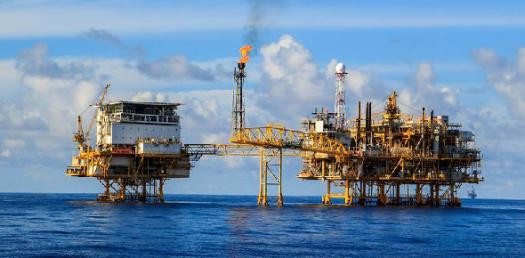# Gases Quiz 1

10 Questions | Attempts: 1531SettingsGas quiz 1

• 1.
A glass column is filled with mercury and inverted in a pool of mercury.  The mercury column stabilizes at a height of 735 mm above the pool of mercury.  What is the pressure of the atmosphere?
• A.

0.697 atm

• B.

0.735 atm

• C.

0.967 atm

• D.

1.03 atm

• E.

194 atm

• 2.
Consider three one-liter flasks at STP.  Flask A contains NH3 gas, flask B contains NO2 gas and flask C contains N2 gas.  Which contains the largest number of molecules
• A.

• B.

• C.

• D.

All are the same

• E.

None

• 3.
Consider three one-liter flasks at STP.  Flask A contains NH3 gas, flask B contains NO2 gas and flask C contains N2 gas.  In which flask are the molecules least polar and therefore most ideal in behavior?
• A.

• B.

• C.

• D.

All are the same

• E.

None

• 4.
Consider three one-liter flasks at STP.  Flask A contains NH3 gas, flask B contains NO2 gas and flask C contains N2 gas.   In which flask do the molecules have the highest average velocity?
• A.

• B.

• C.

• D.

All are the same

• E.

None

• 5.
Consider three one-liter flasks labeled A, B, and C filled with the gases NO, NO2, and N2O, respectively, each at STP.   In which flask do the molecules have the highest average kinetic energy?
• A.

• B.

• C.

• D.

None

• E.

All are the same

• 6.
Consider three one-liter flasks labeled A, B, and C filled with the gases NO, NO2, and N2O, respectively, each at STP.  Which flask contains the fewest ATOMS?
• A.

• B.

• C.

• D.

None

• E.

All are the same

• 7.
Consider three one-liter flasks labeled A, B, and C filled with the gases NO, NO2, and N2O, respectively, each at STP.  Which flask contains 1.0 mole of gas?
• A.

• B.

• C.

• D.

None

• E.

All are the same

• 8.
Consider three one-liter flasks labeled A, B, and C filled with the gases NO, NO2, and N2O, respectively, each at STP.  Which flask contains dinitrogen oxide?
• A.

• B.

• C.

• D.

None

• E.

All are the same

• 9.
Three 1.00 L flasks at 25oC and 725 torr contain the gases CH4 (flask A), CO2 (flask B), and C2H6 (flask C).   In which flask is there 0.039 mole of gas?
• A.

• B.

• C.

• D.

All

• E.

None

• 10.
Three 1.00 L flasks at 25oC and 725 torr contain the gases CH4 (flask A), CO2 (flask B), and C2H6 (flask C).   In which single flask do the molecules have the greatest mass, the greatest average velocity, AND the highest kinetic energy?
• A.

• B.

• C.

• D.

All

• E.

None

## Related TopicsBack to top
×

Wait!
Here's an interesting quiz for you.